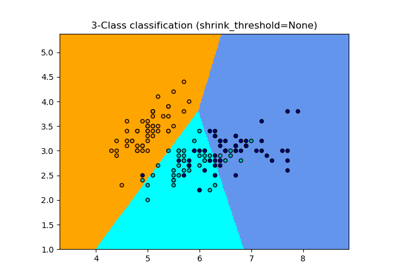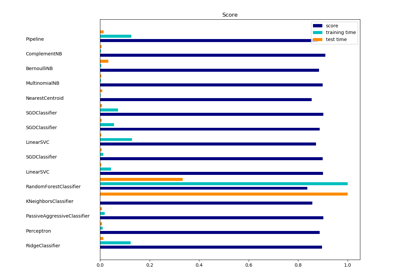# sklearn.neighbors.NearestCentroid¶

class sklearn.neighbors.NearestCentroid(metric='euclidean', shrink_threshold=None)[source]

Nearest centroid classifier.

Each class is represented by its centroid, with test samples classified to the class with the nearest centroid.

Read more in the User Guide.

Parameters
metricstring, or callable

The metric to use when calculating distance between instances in a feature array. If metric is a string or callable, it must be one of the options allowed by metrics.pairwise.pairwise_distances for its metric parameter. The centroids for the samples corresponding to each class is the point from which the sum of the distances (according to the metric) of all samples that belong to that particular class are minimized. If the “manhattan” metric is provided, this centroid is the median and for all other metrics, the centroid is now set to be the mean.

shrink_thresholdfloat, optional (default = None)

Threshold for shrinking centroids to remove features.

Attributes
centroids_array-like of shape (n_classes, n_features)

Centroid of each class.

classes_array of shape (n_classes,)

The unique classes labels.

sklearn.neighbors.KNeighborsClassifier

nearest neighbors classifier

Notes

When used for text classification with tf-idf vectors, this classifier is also known as the Rocchio classifier.

References

Tibshirani, R., Hastie, T., Narasimhan, B., & Chu, G. (2002). Diagnosis of multiple cancer types by shrunken centroids of gene expression. Proceedings of the National Academy of Sciences of the United States of America, 99(10), 6567-6572. The National Academy of Sciences.

Examples

>>> from sklearn.neighbors import NearestCentroid
>>> import numpy as np
>>> X = np.array([[-1, -1], [-2, -1], [-3, -2], [1, 1], [2, 1], [3, 2]])
>>> y = np.array([1, 1, 1, 2, 2, 2])
>>> clf = NearestCentroid()
>>> clf.fit(X, y)
NearestCentroid()
>>> print(clf.predict([[-0.8, -1]]))



Methods

 fit(self, X, y) Fit the NearestCentroid model according to the given training data. get_params(self[, deep]) Get parameters for this estimator. predict(self, X) Perform classification on an array of test vectors X. score(self, X, y[, sample_weight]) Return the mean accuracy on the given test data and labels. set_params(self, \*\*params) Set the parameters of this estimator.
__init__(self, metric='euclidean', shrink_threshold=None)[source]

Initialize self. See help(type(self)) for accurate signature.

fit(self, X, y)[source]

Fit the NearestCentroid model according to the given training data.

Parameters
X{array-like, sparse matrix} of shape (n_samples, n_features)

Training vector, where n_samples is the number of samples and n_features is the number of features. Note that centroid shrinking cannot be used with sparse matrices.

yarray, shape = [n_samples]

Target values (integers)

get_params(self, deep=True)[source]

Get parameters for this estimator.

Parameters
deepbool, default=True

If True, will return the parameters for this estimator and contained subobjects that are estimators.

Returns
paramsmapping of string to any

Parameter names mapped to their values.

predict(self, X)[source]

Perform classification on an array of test vectors X.

The predicted class C for each sample in X is returned.

Parameters
Xarray-like of shape (n_samples, n_features)
Returns
Cndarray of shape (n_samples,)

Notes

If the metric constructor parameter is “precomputed”, X is assumed to be the distance matrix between the data to be predicted and self.centroids_.

score(self, X, y, sample_weight=None)[source]

Return the mean accuracy on the given test data and labels.

In multi-label classification, this is the subset accuracy which is a harsh metric since you require for each sample that each label set be correctly predicted.

Parameters
Xarray-like of shape (n_samples, n_features)

Test samples.

yarray-like of shape (n_samples,) or (n_samples, n_outputs)

True labels for X.

sample_weightarray-like of shape (n_samples,), default=None

Sample weights.

Returns
scorefloat

Mean accuracy of self.predict(X) wrt. y.

set_params(self, **params)[source]

Set the parameters of this estimator.

The method works on simple estimators as well as on nested objects (such as pipelines). The latter have parameters of the form <component>__<parameter> so that it’s possible to update each component of a nested object.

Parameters
**paramsdict

Estimator parameters.

Returns
selfobject

Estimator instance.

## Examples using sklearn.neighbors.NearestCentroid¶